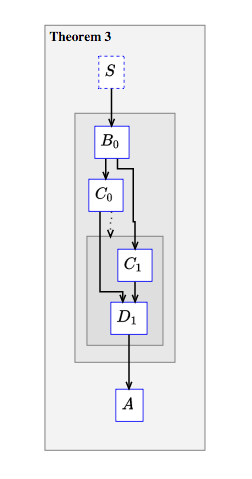# Subdeductions#

Often a proof $$P$$ contains a subargument $$S$$ that stands almost or completely on its own. It may be that $$S$$ was included in $$P$$ because it was not important enough to be stated as its own lemma, or $$S$$ may depend on some assertions, definitions, or assumptions arising earlier in $$P$$. For a subproof like this, Proofscape offers a feature called a subdeduction.

A subdeduction is a special node, of type subdeduc. It is declared and given a name just like any other node. It does not get a preamble, like a proper deduction would, but its internal structure is the same as that of a proper deduction: node definitions, followed by a Meson script.

Listing 12 Subdeduc format#
subdeduc NAME {
NODE_DEFINITIONS
MESON_SCRIPT
}


Like all other compound nodes (such as Existential nodes and Relation chain nodes), a subdeduction itself can be named in a Meson script, and so can its internal nodes, using dotted paths.

Conversely, if $$S$$ is any deduction – either a proper deduction or a subdeduction – and if $$T$$ is a subdeduction declared within $$S$$, then the Meson script of $$T$$ can refer to nodes in $$S$$, according to the usual rules of scopes in programming languages.

For example if both $$S$$ and $$T$$ declare a node called A10, then the Meson script of $$T$$ can refer to the node in $$T$$ as A10 and that of $$S$$ as S.A10. If, on the other hand, $$S$$ declares a node called I20 but $$T$$ does not, then in the Meson script for $$T$$ this node can be referenced simply as I20 (or as S.I20 – they both work). In other words,

A subdeduction defines a new scope within another deduction. As in most programming languages, names (of both nodes and deductions) defined in wider scopes are available within narrower scopes unless overridden.

For example, in Listing 13 the deduction Pf features a subdeduction named SD1. In the Meson script for the subdeduction, B refers to the node named B in Pf, whereas C refers to the node named C in SD1. Meanwhile Pf.C refers to the node by the same name in Pf.

Listing 13 The proof features a subdeduc#
deduc Thm {
supp S {
sy="$S$"
}
asrt A {
sy="$A$"
}
meson="Suppose S. Then A."
}

deduc Pf of Thm.A {

asrt B {
sy="$B_0$"
}

asrt C {
sy="$C_0$"
}

subdeduc SD1 {
asrt C {
sy="$C_1$"
}
asrt D {
sy="$D_1$"
}
meson="
From B get C.
Then D, using Pf.C.
"
}

meson="
From Thm.S get B. Therefore C.
Now SD1.
From SD1.D get Thm.A.
"
}Fig. 13 Proof featuring a subdeduc#